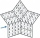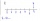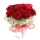# Divisibility + reason - math problems

#### Number of problems found: 105

• Lcm = 22 + gcdThe least common multiple of two numbers is 22 more than their greatest common divisor. Find these numbers.
• We rollWe roll two dice A. - what is the probability that the sum of the falling numbers is at most 4 B. - is at least 10 C. - is divisible by 5?
• Five-digit numberAnna thinks of a five-digit number that is not divisible by three or four. If he increments each digit by one, it gets a five-digit number that is divisible by three. If he reduces each digit by one, he gets a five-digit number divisible by four. If it sw
• TogetherThe three friends divided the balls in a ratio of 6: 5: 4. Some two of them got a total of 126 balls. How many balls were there together?
• Coloured numbersMussel wrote four different natural numbers with coloured markers: red, blue, green and yellow. When the red number divides by blue, it gets the green number as an incomplete proportion, and yellow represents the remainder after this division. When it div
• All pairsDetermine all pairs (m, n) of natural numbers for which is true: m s (n) = n s (m) = 70, where s (a) denotes the digit sum of the natural number a.
• Divisible by nineHow many three-digit natural numbers in total are divisible without a remainder by the number 9?
• How manyHow many numbers are less than 222 with a digit sum is 8?
• Air draftThe numbers 1,2,3,4,5 are written on five tickets on the table. Air draft randomly shuffled the tickets and composed a 5-digit number from them. What is the probability that he passed: and, the largest possible number b, the smallest possible number c, a
• Columns of two and threeWhen students in one class stand in columns of two, there is none left. When he stands in columns of three, there is one student left. There are 5 more double columns than three columns. How many students are in the class?
• Four-digit numbersFind four-digit numbers where all the digits are different. For numbers, the sum of the third and fourth digits is twice the sum of the first two digits, and the sum of the first and fourth digits is equal to the sum of the second and third digits. The di
• ProbabilityHow probable is a randomly selected three-digit number divisible by five or seven?
• The floristA consignment of 200 roses arrived at the florist in the morning. She sold more than half of them during the day. She wants to tie bouquets of the remaining roses. If she ties a bouquet of three, four, five, or six roses, there will always be one rose lef
• Integer cubeThe length of the cube edge is an integer. Its volume is in cm3 a five-digit number divisible by 1331. What is the length of the edge of this cube.
• Twenty-fiveHow many are three-digit natural numbers divisible by 25?
• Intelligence testPaľo, Jano, Karol, and Rišo were doing an intelligence test. Palo correctly answered half of the questions plus 7 questions, Jano to a third plus 18 questions, Karol to a quarter plus 21 questions and Risho to a fifth plus 25 questions. After the test, Ka
• By sixFrom the digits 1,2,3,4 we create the long integer number 123412341234. .. .. , which will have 962 digits. Is this number divisible by 6?
• Sum of the digitsHow many are two-digit natural numbers that have the sum of the digits 9?
• Math testIn mathematics, there were 25 problems of three kinds: light 2 points, medium 3 points, heavy 5 points, the best score is 84 points. How many points did Jane have when she solved all the easy examples, half medium and one-third difficult?
• NumberWhat number should be placed instead of the asterisk in number 702*8 to get a number divisible by 6?

Do you have an exciting math question or word problem that you can't solve? Ask a question or post a math problem, and we can try to solve it.

We will send a solution to your e-mail address. Solved examples are also published here. Please enter the e-mail correctly and check whether you don't have a full mailbox.

Please do not submit problems from current active competitions such as Mathematical Olympiad, correspondence seminars etc...

Divisibility - math problems. Reason - math problems.